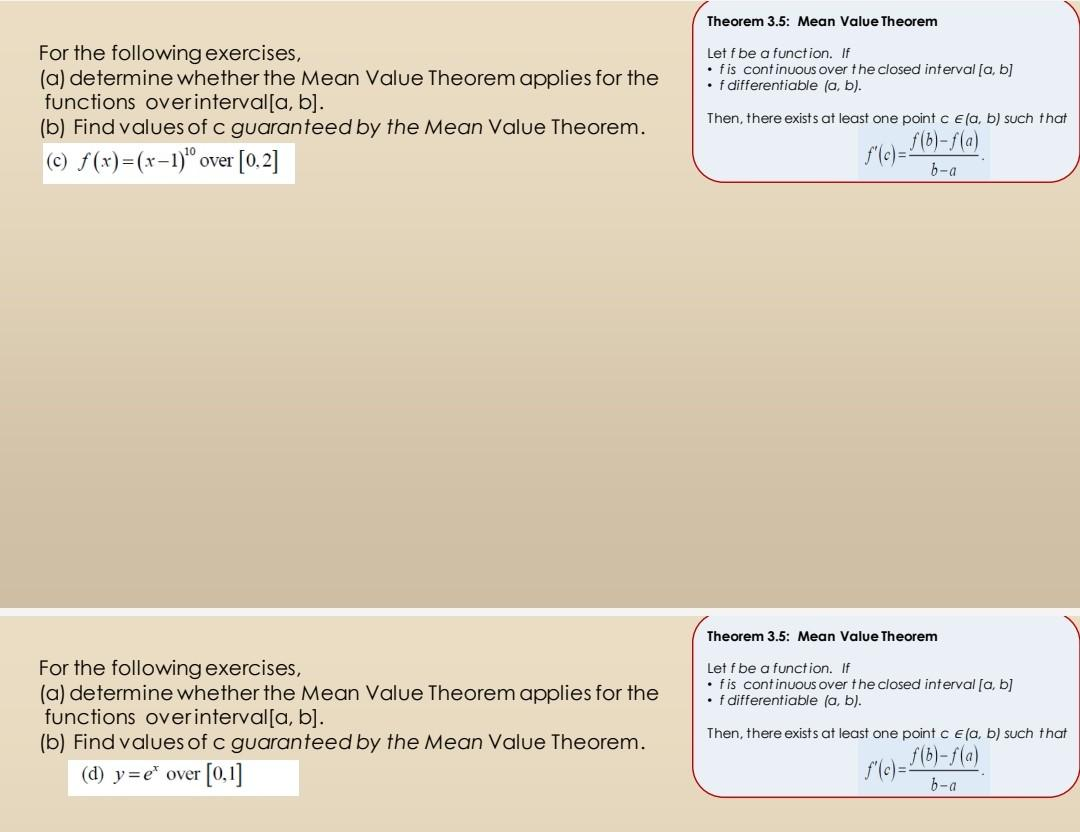# Question Solved1 Answer For the following exercises, (a) determine whether the Mean Value Theorem applies for the functions over interval[a, b]. (b) Find values of c guaranteed by the Mean Value Theorem. (c) f(x)=(x-1)¹0 over [0.2] For the following exercises, (a) determine whether the Mean Value Theorem applies for the functions over interval[a, b]. (b) Find values of c guaranteed For the following exercises, (a) determine whether the Mean Value Theorem applies for the functions over interval[a, b]. (b) Find values of c guaranteed by the Mean Value Theorem. (c) f(x)=(x-1)¹0 over [0.2] For the following exercises, (a) determine whether the Mean Value Theorem applies for the functions over interval[a, b]. (b) Find values of c guaranteed by the Mean Value Theorem. (d) y=e¹ over [0,1] Theorem 3.5: Mean Value Theorem Let f be a function. If • fis continuous over the closed interval [a, b] •f differentiable (a, b). Then, there exists at least one point c e(a, b) such that f'(c)=f(b)-f(a) b-a Theorem 3.5: Mean Value Theorem Let f be a function. If • fis continuous over the closed interval [a, b] • f differentiable (a, b). Then, there exists at least one point c e(a, b) such that f'(c)=f(b)-f(a) b-aTranscribed Image Text: For the following exercises, (a) determine whether the Mean Value Theorem applies for the functions over interval[a, b]. (b) Find values of c guaranteed by the Mean Value Theorem. (c) f(x)=(x-1)¹0 over [0.2] For the following exercises, (a) determine whether the Mean Value Theorem applies for the functions over interval[a, b]. (b) Find values of c guaranteed by the Mean Value Theorem. (d) y=e¹ over [0,1] Theorem 3.5: Mean Value Theorem Let f be a function. If • fis continuous over the closed interval [a, b] •f differentiable (a, b). Then, there exists at least one point c e(a, b) such that f'(c)=f(b)-f(a) b-a Theorem 3.5: Mean Value Theorem Let f be a function. If • fis continuous over the closed interval [a, b] • f differentiable (a, b). Then, there exists at least one point c e(a, b) such that f'(c)=f(b)-f(a) b-a
More
Transcribed Image Text: For the following exercises, (a) determine whether the Mean Value Theorem applies for the functions over interval[a, b]. (b) Find values of c guaranteed by the Mean Value Theorem. (c) f(x)=(x-1)¹0 over [0.2] For the following exercises, (a) determine whether the Mean Value Theorem applies for the functions over interval[a, b]. (b) Find values of c guaranteed by the Mean Value Theorem. (d) y=e¹ over [0,1] Theorem 3.5: Mean Value Theorem Let f be a function. If • fis continuous over the closed interval [a, b] •f differentiable (a, b). Then, there exists at least one point c e(a, b) such that f'(c)=f(b)-f(a) b-a Theorem 3.5: Mean Value Theorem Let f be a function. If • fis continuous over the closed interval [a, b] • f differentiable (a, b). Then, there exists at least one point c e(a, b) such that f'(c)=f(b)-f(a) b-a